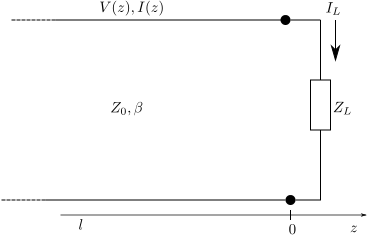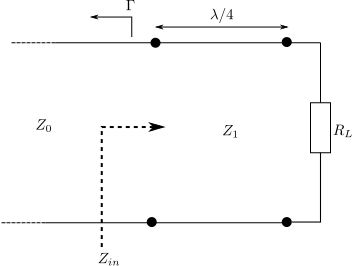- DSP log - http://www.dsplog.com -

GATE-2012 ECE Q28 (electromagnetics)

Posted By Krishna Sankar On February 20, 2013 @ 6:50 am In GATE | No Comments

Question 28 on electromagnetics from GATE (Graduate Aptitude Test in Engineering) 2012 Electronics and Communication Engineering paper.

## (D) 1.75m## Solution

To answer this question, let us first understand the propagation in a transmission line,  termination and the concept of impedance matching. The section 2.1 in Microwave Engineering, David M Pozar (buy from Amazon.com, Buy from Flipkart.com  is used as reference.

Consider a transmission line of very small length  having the parameters as show in figure below.Figure : Transmission line model  (Reference Figure 2.1 in Microwave Engineering, David M Pozar (buy from Amazon.comBuy from Flipkart.com )

is the resistance per unit length ,

is the inductance per unit length ,

is the conductance per unit length ,

is the capacitance per unit length.

Applying Kirchoff’s voltage law,

Applying Kirchoff’s current law,

Dividing the above equations by  and taking the limit ,

If we assume that the inputs are sinusoidal, then the above equation can be re-written as

Substituting,

,

,

where

.

The solution to the above equations are,

.

The current on the line can be alternately expressed as,

,

where the characteristic impedance of the line is defined as,

.

The wavelength on the line is,

.

Loss less transmission line case

For a lossless transmission line, we can set .

Then the propagation constant reduces to, the characteristic impedance is  and the voltage and current on the line can be written as,

## Terminated lossless transmission line

Consider a transmission line terminated with load impedance  as shown in figure below.Figure: Transmission line with load impedance (Reference Figure 2.4 in Microwave Engineering, David M Pozar (buy from Amazon.comBuy from Flipkart.com )

At the load , the relation between the total voltage and current is related to the load impedance

.

Alternatively,

.

The reflection coefficient  is defined as the amplitude of the reflected voltage to the incident voltage,

.

For no reflection to happen, i.e , the load impedance should be equal to the characteristic impedance of the transmission line. The above equation captures the impedance seen at the load

The voltage and current on the line can be represented using  as,

When looking from a point  from the load, the input impedance seen is,

.

Substituting for

.

Special case when  (and it’s odd multiples)

For the case when  the input impedance seen is,

.

This result can be used to for impedance matching.

## Quarter wave transformer

Consider a circuit with load  and a line with characteristic impedance connected by a transmission line of characteristic impedance with length .Figure: Quarter Wave Matching transformer (Reference Figure 2.16 in Microwave Engineering, David M Pozar (buy from Amazon.comBuy from Flipkart.com )

The input impedance seen is,

.

So if we choose , then the input impedance seen is  which is the condition required for having no reflection i.e. .

One important aspect to note here is that  is not guaranteed for all frequencies, but rather only for certain frequencies. The frequency dependence can be found by finding the frequencies for which  .

Replacing  where is the wave length corresponding to frequency ,

.

It can be seen that only for  , the term resulting in reflection coefficient only for those frequencies.

## Solving the GATE question

Applying all this to the problem at hand, we have   and .

Given that , we know that a quarter wave transformer is used to achieve impedance matching.

Now we also know that we need to match for two frequencies  and .

The wavelength for each frequencies are,

The least common multiple of these two wavelength is,  and the corresponding quarter wave length is .

Given than  is not listed in the options, we can go for the next higher odd multiple i.e.

Based on the above, the right choice is (C) 1.58m

## References

 GATE Examination Question Papers [Previous Years] from Indian Institute of Technology, Madras http://gate.iitm.ac.in/gateqps/2012/ec.pdf 

 Microwave Engineering, David M Pozar (buy from Amazon.comBuy from Flipkart.com 

URL to article: http://www.dsplog.com/2013/02/20/gate-2012-ece-q28-electromagnetics/

URLs in this post: